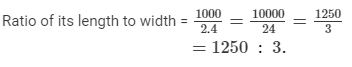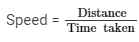Courses

# RD Sharma Solutions -Page No.9.5 & 9.6, Ratio Proportion And Unitary Method, Class 6, Maths Class 6 Notes | EduRev

## RD Sharma Solutions for Class 6 Mathematics

Created by: Abhishek Kapoor

## Class 6 : RD Sharma Solutions -Page No.9.5 & 9.6, Ratio Proportion And Unitary Method, Class 6, Maths Class 6 Notes | EduRev

The document RD Sharma Solutions -Page No.9.5 & 9.6, Ratio Proportion And Unitary Method, Class 6, Maths Class 6 Notes | EduRev is a part of the Class 6 Course RD Sharma Solutions for Class 6 Mathematics.
All you need of Class 6 at this link: Class 6

#### PAGE NO 9.5:

Question 1: Express each of the following in the language of ratios:
(i) In a class, the number of girls in the merit list of the board examination is two times that of boys.
(ii) The number of student passing mathematics test is 2/3 of the number that appeared.

(i)  The ratio of the number of girls in the merit list of the board examination to the number of boys in that list is 2 : 1.

(ii) The ratio of the number of students passing mathematics test to the total number of students appeared is 2 : 3.

#### PAGE NO 9.6:

Question 2: Express the following ratios in language of daily life:
(i) The ratio of the number of bad pencils to that good pencils produced in a factory is 1:9.
(ii) In India, the ratio of the number of villages to that of cities is about 2000:1.

ANSWER: (i) The ratio of the number of bad pencils to that of good pencils produced in a factory is 1 : 9 means out of 10 or (9 + 1)  pencils, 9 pencils are good and 1 is bad.
(ii) In India, the number of villages is 2,000 times that of the cities.

Question 3: Express each of the of the following rations in its simplest from:

(i) 60:72
(ii) 324:144
(iii) 85:391
(iv) 186:403

ANSWER: (i) 60 : 72 = 5 : 6 (dividing by 12)
(ii) 324 : 144 = 9 : 4 (dividing by 36)
(iii) 85 : 391 = 5 : 23 (dividing by 17)
(iv) 186 : 403 = 6 : 13 (dividing by 31)

Question 4: Find the ratio of the following in the simplest form:

(i) 75 paisa to Rs 3
(ii) 35 minutes to 45 minutes
(iii) 8 kg to 400 gm
(iv) 48 minutes to 1 hour
(v) 2 metres to 35 cm
(vi) 35 minutes to 45 seconds
(vii) 2 dozen to 3 scores
(viii) 3 weeks to 3 days
(ix) 48 min to 2 hour 40 min
(x) 3 m 5 cm to 35 cm.

(i) ∵ Rs. 1 = 100 paise
∴ 75  : 300 = 1 : 4

(ii)  35 : 45 = 7 : 9
(iii) ∵ 1 kg = 1000 gm
∴ 8000 : 400 = 20 : 1
(iv) ∵ 1 Hour = 60 minute
∴48 : 60 = 4 : 5
(v) ∵ 1 meter = 100 cm
∴ 200 : 35 = 40 : 7
(vi) ∵ 1 min = 60 sec.
∴ 35×60 : 45 = 140 : 3
(vii) ∵ 1 dozen = 12
∴ 1 score = 20
or 3 score = 3 × 20 = 60
∴ 24 : 60 = 2 : 5.
(viii) ∵ 1 week = 7 days
∴ 21 : 3 = 7 : 1
(ix) ∵ 1 hour = 60 minute
∴ 2 hour 40 min = 2 × 60 + 40 = 160
∴ 48 : 160 = 3 : 10
(x) ∵ 1 meter = 100 cm
∴ 3 m 5 cm = 3 ×100 + 5 = 305 cm
∴ 305 : 35 = 61 : 7

Question 5: Find the ratio of

(i) 3.2 metres to 56 metres
(ii) 10 metres to 25 cm.
(iii) 25 paisa to Rs 60
(iv) 10 litres to 0.25 litre

ANSWER: (i) The ratio of 3.2 metres to 56 metres = 3.2 : 56 = 0.4 : 7 = 2 : 35
(ii) The ratio of 10 metres to 25 cm = 1000 : 25 = 40 : 1
(iii) The ratio of 25 paisa to Rs 60 = 25 : 6000 = 1 : 240
(iv) The ratio of 10 litres to 0.25 litre = 10 : 0.25 = 40 : 1

Question 6: The number of boys and girls in a school are 1168 and 1095 respectively. Express the ratio of the number of boys to that of the girls in the simplest form.

ANSWER: Ratio of the number of boys to that of the girls is 1168 : 1095 = 16 : 15 (dividing by 73)

Question 7: Avinash works as a lecturer and earns Rs 12000 per month. His wife who is a doctor earns Rs 15000 per month. Find the following ratios:

(i) Avinash's income to the income of his wife
(ii) Avinash's income to their total income.

ANSWER: Avinash's income = Rs. 12000
Avinash's wife income = Rs. 15000
Total income = 12000 + 15000 = Rs. 27000
(i) Ratio of Avinash's income to the income of his wife = 12000 : 15000 = 4 : 5
(ii) Ratio of Avinash's income to their total income = 12000 : 27000 = 4 : 9

Question 8: Of the 72 persons working in an office, 28 are men and the remaining are women. Find the ratio of the number of:

(i) men to that of women
(ii) men to the total number persons
(iii) persons to that of women.

Total number of persons = 72
Number of men = 28
Number of women = 72 −- 28 = 44
(i) Ratio of men to that of women = 28 : 44 = 7 : 11
(ii) Ratio of men to the total number of persons = 28 : 72 = 7 : 18
(iii) Ratio of persons to that of women = 72 : 44 = 18 : 11

Question 9: The length of a steel tape for measurements of buildings is 10 m and its width is 2.4 cm. What is the ratio of its length to width?

Length of the steel tape = 10 m
= 10×100 cm10×100 cm = 1000 cm
Breadth of the steel tape = 2.4 cmQuestion 10: An office opens at 9 a.m. and closed at 5 p.m. with a lunch interval of 30 minutes. What is the ratio of lunch interval to the total period in office?

Total period in office = 9 a.m. to 12 p.m. and 12 p.m. to 5 p.m.
= 3 hours + 5 hours = 8 hours
∵ 1 hour = 60 minutes
∴ 8 hours = 8 × 60 = 480 minutes
Ratio of lunch interval to total period = 30 : 480 = 1 : 16 (dividing by 30)

Question 11: A bullock-cart travels 24 km in 3 hours and a train travels 120 km in 2 hours. Find the ratio fo their speeds.Distance covered by bullock-cart = 24 km
Time taken = 3 hours
Speed of bullock-cart = 24/3=8 km/h

Distance covered by train = 120 km
Time taken = 2 hours
Speed of train = 120/2=60 km/h

Ratio for their speeds = Speed of bullock-cart : Speed of train = 8 : 60
= 2 : 15

Question 12: Margarette works in a factory and earns Rs 955 per month. She saves Rs 185 per month from her earnings. Find the ratio of:

(i) her savings to her income
(ii) her income to her expenditure
(iii) her savings to her expenditure.

Income of Margarette per month = Rs. 955
Saving of Margarette per month = Rs. 185
Expenditure of Margarette per month = 955−-185 = Rs. 770
(i) Ratio of her saving to her income = 185 : 955 = 37 : 191
(ii) Ratio of her income to her expenditure = 955 : 770 =  191 : 154
(iii) Ratio of her saving to her expenditure = 185 : 770 =  37 : 154

(i)  Rs. 1 = 100 paise      75  : 300 = 1 : 4(ii)  35 : 45 = 7 : 9(iii)  1 kg = 1000 gm     8000 : 400 = 20 : 1(iv)  1 Hour = 60 minute     48 : 60 = 4 : 5(v)  1 meter = 100 cm     200 : 35 = 40 : 7(vi)  1 min = 60 sec.      35×60 : 45 = 140 : 3(vii)  1 dozen = 12       1 score = 20   or 3 score = 3 × 20 = 60    24 : 60 = 2 : 5.(viii)  1 week = 7 days       21 : 3 = 7 : 1(ix)  1 hour = 60 minute      2 hour 40 min = 2 × 60 + 40 = 160      48 : 160 = 3 : 10(x)  1 meter = 100 cm      3 m 5 cm = 3 ×100 + 5 = 305 cm     305 : 35 = 61 :

103 docs

,

,

,

,

,

,

,

,

,

,

,

,

,

,

,

,

,

,

,

,

,

,

,

,

,

,

,

,

,

,

;